### The Network Simplex Algorithm

The network simplex solver in PROC OPTMODEL attempts to leverage the speed of the network simplex algorithm to more efficiently solve linear programs by using the following process:

1. It heuristically extracts the largest possible network substructure from the original problem.

2. It uses the network simplex algorithm to solve for an optimal solution to this substructure.

3. It uses this solution to construct an advanced basis to warm-start either the primal or dual simplex solver on the original linear programming problem.

The network simplex algorithm is a specialized version of the simplex algorithm that uses spanning-tree bases to more efficiently solve linear programming problems that have a pure network form. Such LPs can be modeled using a formulation over a directed graph, as a minimum-cost flow problem. Let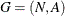be a directed graph, where N denotes the nodes and A denotes the arcs of the graph. The decision variable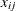denotes the amount of flow sent between node i and node j. The cost per unit of flow on the arcs is designated by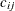, and the amount of flow sent across each arc is bounded to be within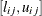. The demand (or supply) at each node is designated as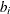, where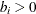denotes a supply node and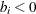denotes a demand node. The corresponding linear programming problem is as follows: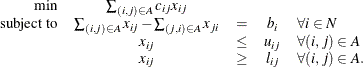The network simplex algorithm used in PROC OPTMODEL is the primal network simplex algorithm. This algorithm finds the optimal primal feasible solution and a dual solution that satisfies complementary slackness. Sometimes the directed graph G is disconnected. In this case, the problem can be decomposed into its weakly connected components, and each minimum-cost flow problem can be solved separately. After solving each component, the optimal basis for the network substructure is augmented with the non-network variables and constraints from the original problem. This advanced basis is then used as a starting point for the primal or dual simplex method. The solver automatically selects the solver to use after network simplex. However, you can override this selection with the ALGORITHM2= option.

The network simplex algorithm can be more efficient than the other solvers on problems that have a large network substructure. The size of this network structure can be seen in the log.